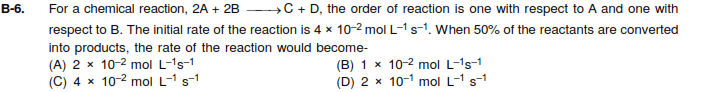• sidt36

## Homework Statement## Homework Equations

Is the question asking the concentration when the system has reached its half life
Can use the half life formula?[/B]

## The Attempt at a Solution

So

1/cT + 1/co = kt

1/(4 *10-2) + 1/x = k(half life time)

Is this the right approach

The question is slightly ambiguous. I guess, but it is not explicitly stated, that the initial concentrations of A and B are the same.

So then you have in practice second order kinetics with respect to A, for there A = B at all times. So practically you would have the second or the kinetics with respect to A (or B).

There is a formula for half-life with second order kinetics but I don't think it's the one you have used.

Best to try and work this out from first principles. Then look it up in any case. That is the second part of this test. But the first part – knowing that this was what you had to do was the more important olympiadic part.

I never remember very well this formula - reason is there is not commonly all that much use for it outside of Olympiadics, at least in my experience. I just remember there is such a formula, and can always work it out from first principles.

Well I Have a solution with some logic (...)
R α CA CB

When we half each concentration the Total rate falls by ¼
So the answer should be 1×102

Yes that is a good way to reason.

Notice that it did depend on the initial [A] = [ B]

(10-2 btw).

Last edited: